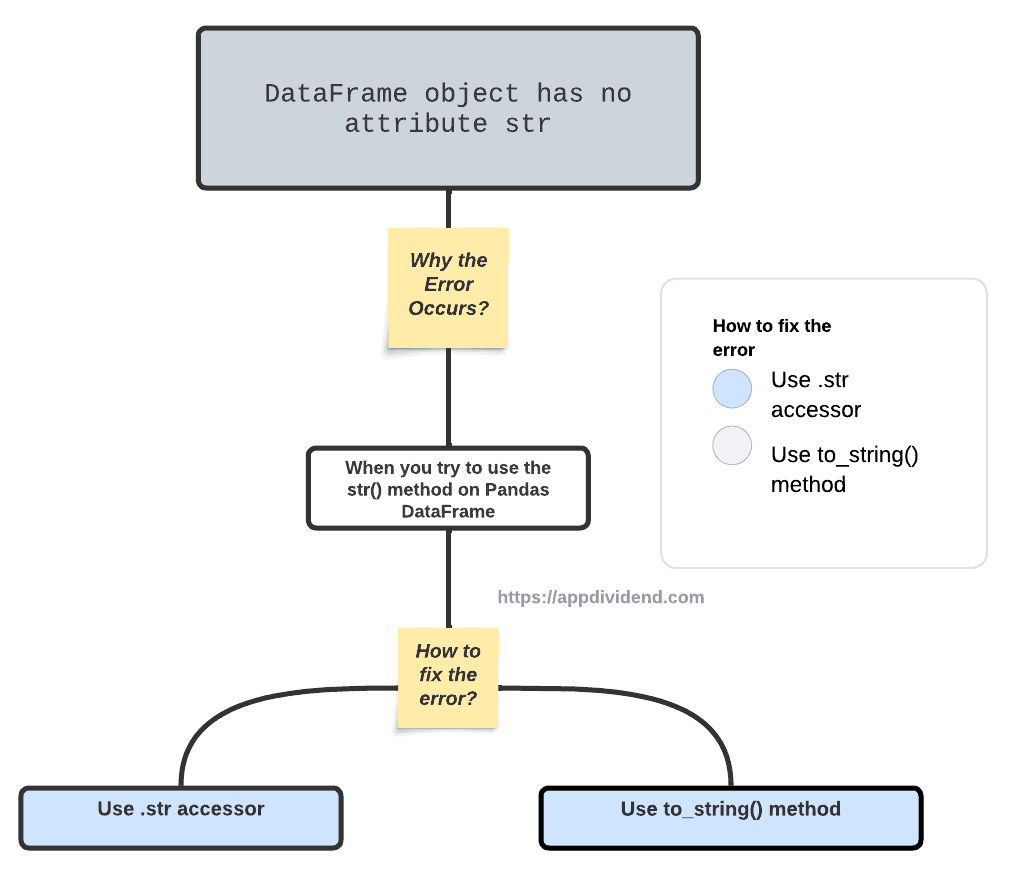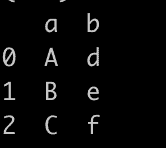# How to Fix AttributeError: ‘DataFrame’ object has no attribute ‘str’Diagram

AttributeError: ‘DataFrame’ object has no attribute ‘str’ occurs when you try to “use the str() method on a Pandas DataFrame.” The str() method is used to convert an object to a string representation, but it is not defined for DataFrames.

The str() method is only defined for Series objects. A Series is a one-dimensional Pandas object, while a DataFrame is a two-dimensional Pandas object. A Series can be represented as a single string, while a DataFrame cannot.

To fix the AttributeError: ‘DataFrame’ object has no attribute ‘str’ error:

1. Use the “.str” accessor on the Series object
2. Use the “to_string” method

## Reproduce the error

``````import pandas as pd

df = pd.DataFrame({'a': [1, 2, 3], 'b': [4, 5, 6]})

print(df.str)
``````

Output

``AttributeError: 'DataFrame' object has no attribute 'str'``

## How to fix it?

### Solution 1: Using the “.str” accessor on the DataFrame column

``````import pandas as pd

df = pd.DataFrame({'a': ["A", "B", "C"], 'b': ["D", "E", "F"]})

df['b'] = df['b'].str.lower()

````print(df)````

Output### Solution 2: Using the to_string() method

The to_string() method is “used to convert a DataFrame to a string representation.”

``````import pandas as pd

df = pd.DataFrame({'a': ["A", "B", "C"], 'b': ["D", "E", "F"]})

df = df.to_string().lower()

print(df)
``````

Output

``````   a   b
0  a   d
1  b   e
2  c   f
``````

The to_string() method will print the DataFrame in a human-readable format. It will also include the column names and the index.

That’s it! I hope these solutions fix the error.

## Related posts

AttributeError: ‘DataFrame’ object has no attribute ‘map’

AttributeError: ‘DataFrame’ object has no attribute ‘reshape’

AttributeError: ‘DataFrame’ object has no attribute ‘iteritems’

This site uses Akismet to reduce spam. Learn how your comment data is processed.